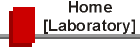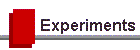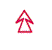##Brief Description of Experiments

This listing includes a brief description of the "free choice" experiments along with the number of weights assigned to each experiment.

EXPLANATION OF NOTATION: The numbers in square brackets following these term indicators, e.g., [1 wt], [2 wt]  indicate the number of weights credited to the experiment. A one weight experiment requires one lab session (3 hours) to complete; a two weight experiment requires two lab sessions (6 hours).

The guide sheets for all of these experiments, (in pdf format) can be viewed and downloaded by returning to the Experiments page and clicking on the links in the table there.

(M) Mechanics and Mechanical Systems

Conservation of Angular Momentum: A series of rings and cylinders are dropped onto a rotating disc and comparisons of the angular momenta before and after the "collisions" are made using a computer interfaced rotational motion sensor. [1 wt]

Free Fall: Measurement of g by determining the distance a body falls in a measured time. [1 wt]

The Gyroscope: A study of this fascinating instrument, in which angular momentum, torque, precession, nutation, etc., can be measured. [1-2 wt]

The Mechanical Equivalent of Heat: Joule's classic experiment. [1wt]

Mechanical Oscillations - Resonance and Ringing in  a Tuning Fork: An investigation of vibrations in a tuning fork subject to damping, using a storage oscilloscope. [1 wt]

Moment of Inertia: The moments of inertia of various objects are determined by applying varying torques to the objects and measuring the corresponding angular accelerations using a computer interfaced angular motion sensor. [1 wt]

Oscillations of a Sphere on a Concave Surface: Measurement of the radius of curvature of a concave surface using a simple harmonic motion system. [1 wt]

The Torsion Pendulum: Measurement of the torsional constant of a wire and the moment of inertia of various solids. [1 wt]

Wilberforce Spring: A fascinating study of mechanical resonance between two types of simple harmonic motion. [1 wt](PM) Properties of Matter

Similarity: The study of the motion of solids in viscous fluids in both laminar and turbulent regimes. Emphasis is placed on considerations involving the changing of scales of the physical quantities. [2 wt]

Surface Tension: Measurement of the surface tension of a number of liquids. Temperature dependence may be studied. [1 wt]

Vapour Pressure of Water: The vapour pressure of water between 300 K and 373 K is studied. An ingenious experiment with several subtleties. [1 wt]

Viscosity of Water by Capillary Flow: Measurement of the coefficient of viscosity of a fluid. [1 wt](W) Wave Phenomena

The Acoustic Interferometer: Study of the interference of sound waves using a classic Michelson-type interferometer. Measures the velocity of sound in air. [1 wt]

Electron Diffraction: Diffraction patterns of pyrolitic graphite and poly-crystalline aluminum can be observed and photographed for measurement; lattice constants may be determined. Required background material may be unfamiliar to you. Preparation is essential. [1 wt]

Interference & Diffraction (Using a Laser): Single and multiple slits are available on photographic plates, and some two-dimensional patterns and gratings for the study of Fraunhofer diffraction. Quantitative comparisons with theory can be made. [1 wt]

Lens Optics: Focusing properties of thin lenses. [1 wt]

Microwave Optics: Wave effects observed using microwaves with a wavelength of 3 cm. A fairly qualitative experiment. [1-2wt]

Optical Activity: Optical Activity refers to the property of some materials, particularly biological ones to rotate the plane of polarization of light waves. You will explore the phenomenon of polarization of light and then study the optical activity of ordinary table sugar (sucrose). [1-2 wt]

Optical Fibers: You will investigate the index of refraction and total internal reflection in a transparent body. You will then study the speed of light propagation in optical fibers of different lengths as well as the attenuation of light in the fiber material. [1wt]

Polarization of light: I. A verification of Malus’ law (the intensity of light transmitted through polarizer and analyzer is measured); II. A study of reflection of polarized light at an air-glass interface. [1-2  wt]

The Speed of Sound in a Solid: Piezoelectric crystals are used to generate and detect sound waves in a rod. The speed of sound in the rod is determined from transit times and also from resonant frequencies of waves reflected from the ends of the rod. [1 wt]

Standing Waves and Acoustic Resonance: A moveable microphone is used to detect the positions of maxima and minima in a standing  sound wave pattern. The speed of sound in air is measured and the quality factor (Q) of the resonant system is determined. [1 wt]

Velocity of Sound in a Pure Gas: Determination of the important constant gamma which is the ratio of the specific heat of a gas at constant pressure to the specific heat at constant volume. [1 wt]

Waves - Steady or Pulsed, Standing or Travelling: An introduction to wave phenomena. Standing and travelling waves are investigated in a torsional wave apparatus, using the aid of your eyes, your hands, an oscilloscope and a signal generator. [1 wt](E) Electric & Electronic Circuits

Current Balance: This experiment defines the ampere. A measurement of current is made in terms of the force between two current carrying conductors. [2 wt]

Digital Electronics parts I & II: In part I, one-transistor amplifiers are combined to make AND gates, binary adders, etc. In part II, TTL Integrated Circuits are used to investigate flip-flops, memories, bistables, etc. [1-2 wt]

The Electrocardiogram: The electric signals from the heart are investigated using and oscilloscope with associated electronics. [1 wt]

Faraday’s Law and the AC Generator: An introduction to the AC generator and several quantitative aspects of Faraday’s Law. [1 wt]

Hall Effect in Thin Films: The transverse voltage produced when a current-carrying conductor is placed in a magnetic field is called the Hall effect. In this experiment the Hall constant is measured for different materials and the charge carriers are identified for those materials. [2 wt]

Thermistors and Diodes: Extending Simple Electric Circuits to study thermistors, diodes, lamps. [1 wt](AN) Atomic and Nuclear Physics

Radioactivity in the Air: A sample of some hundreds of litres of Toronto air is filtered, and the radioactivity collected in the filter is studied. [2 wt]

Scattering: A model of a two-dimensional scattering process. The experiment simulates the scattering of a beam of particles from a fixed target. [1-2 wt]

Spectra: Several sources of emission and absorption spectra are available. The hydrogen spectrum (Balmer series) is studied and the Rydberg constant determined. Gases and mixtures of gases can be identified by study of the spectral lines. [1 wt](F) Fundamental Constants

The Cavendish Experiment: The classic experiment to measure the Universal Gravitational Constant. [2 wt]

e/m for an Electron: Measurement of the charge-to-mass ratio of the electron. The radii of the trajectories of electrons for various values of magnetic field are measured. [1 wt]

The Millikan Oil- Drop Experiment: The classic experiment which shows the quantification of electric charge. [2 wt]

The Speed of Light: A modification of Fizeau’s classic experiment. This is a straightforward but challenging experiment. Great care is needed to set up the apparatus correctly. A careful experimenter can get ±5% results. [2 wt]